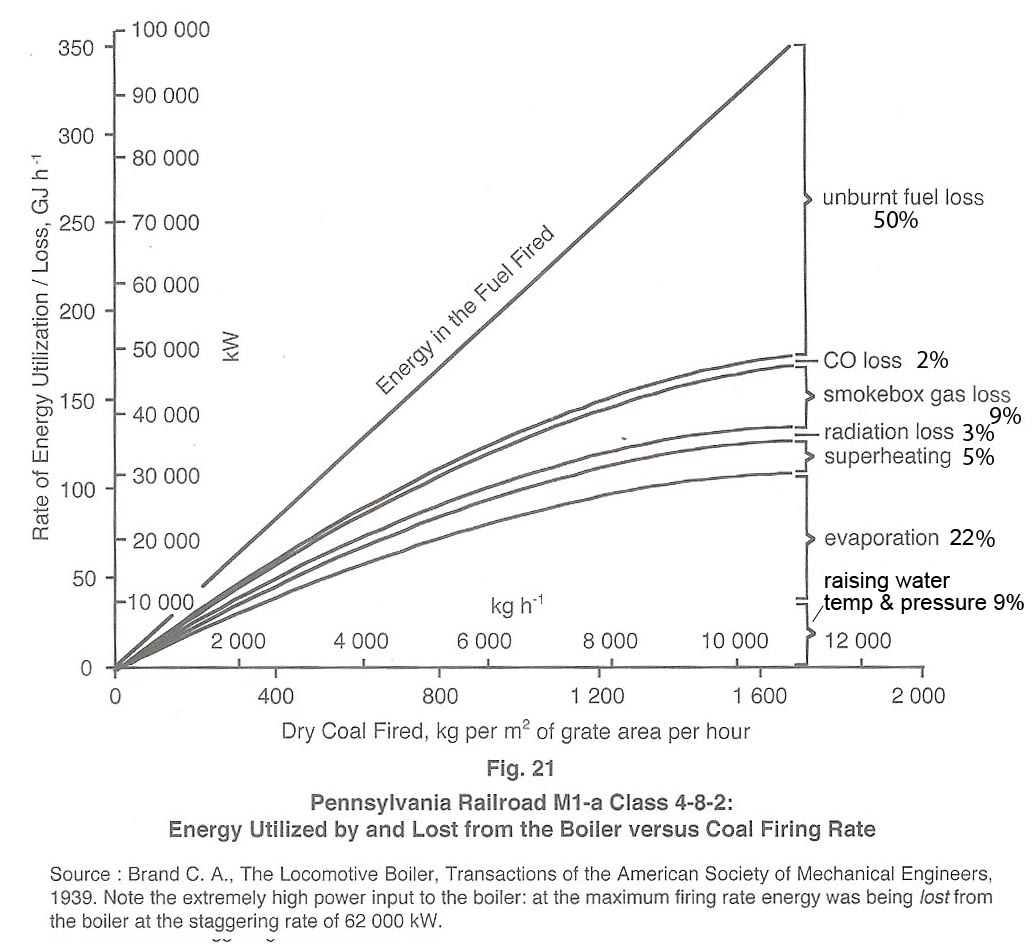# Grate Limit as it relates to Boiler Efficiency

## The Grate Limit as it relates to Boiler Efficiency

On page 78 of his book The Red Devil and Other Tales from the Age of Steam, Dave Wardale defines the Grate Limit for a (normal) locomotive firebox as follows:

The grate limit is the point “at which even by firing more coal and supplying more combustion air, no more steam could be produced.”

Put another way, it is the point at which the rate of firing fuel into the firebox exactly equals the rate at which unburned fuel is carried out of the firebox by entrainment in the combustion air.

Wardale quotes an equation derived by L.H. Fry in 1924 that (effectively) relates the grate limit to boiler efficiency as follows:

Eb = k1 – k2 x M/G, where

• Eb = Boiler Efficiency
• M = Firing Rate
• G = Grate Area
• k1 = predicted boiler efficiency at zero firing rate
• k2 = the slope of the graph relating boiler efficiency to firing rate.

The equation is illustrated in graphical form below (Fig 20 in Wardale’s book):The above equation is empirical, yet it is one that produces a fascinating insight – namely that the boiler efficiency at the grate limit is exactly 50% of the predicted efficiency at zero firing rate.

In the diagram, k1 is the maximum predicted boiler efficiency (at zero firing rate) and k2 is the slope of the straight line relating efficiency to firing rate. [A simple mathematical proof that (based on Fry’s equation) the boiler efficiency at the grate limit is exactly half the maximum efficiency is given further below. A definition of Equivalent Evaporation is also provided on a sepate page.]

Wardale goes on to demonstrate that Fry’s equation represents reality, being demonstrated in a boiler test conducted on a Pennsylvania Railroad M1a 4-8-2 locomotive. Here Wardales adjusts his definition of the grate limit as follows:

The grate limit was the point at which “the heat liberation rate in the firebox was a maximum, which for all practical purposes occurred when the fuel entrained in the draught and escaping unburnt equalled the amount of fuel actually burned, this point being linked to the start of gross firebed fluidisation.”

In other words, the grate limit is reached when half the fuel that is fired into the firebox escapes from the chimney. He illustrates this with the diagram below taken from Fig 21 on page 80 of his book, to which the percentage figures on the right have been added [including an approximate division of “evaporation” into latent and sensible heat]:It should be noted that Fry’s equation does not hold for GPCS fireboxes. Indeed, the fact that it does not hold is one of the great advantages that GPCS fireboxes offer.

A simple mathematical proof that, based on Fry’s equation, boiler efficiency at the grate limit is exactly half the maximum efficiency, is as follows:

Fry’s Equation: Eb = k1 – k2 x M/G, where:

• Eb = Boiler Efficiency
• M = Firing Rate
• G = Grate Area
• k1 = predicted boiler efficiency at zero firing rate
• k2 = the slope of the graph relating boiler efficiency to firing rate.

Boiler efficiency may also be defined as the amount of energy released from the boiler in the form of steam divided by the amount of energy released in the firebox from the fuel.

Thus if the Steaming Rate = S, then Eb = S ÷ M/G

Thus M/G = S/Eb

Substituting this in Fry’s equation we get: Eb = k1 – k2 x S/Eb

from which: Eb2 = k1.Eb – k2.S and thus: S = k1.Eb/k2 – Eb2/k2

From calculus, we know that S reaches a maximum (or minimum) when the slope of the curve = zero. This occurs when$\frac { dS }{ d{ E }_{ b } } =\frac { { k }_{ 1 } }{ { k }_{ 2 } } -\frac { 2{ E }_{ b } }{ { k }_{ 2 } } =0$

i.e. when …..${ E }_{ b } = \frac { { k }_{ 1 } }{ 2 }$

Thus the maximum steaming rate occurs at the point where the boiler efficiency is half the predicted value at zero firing rate.